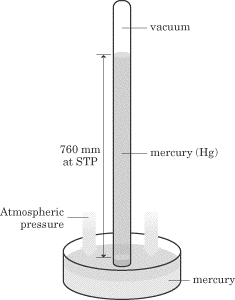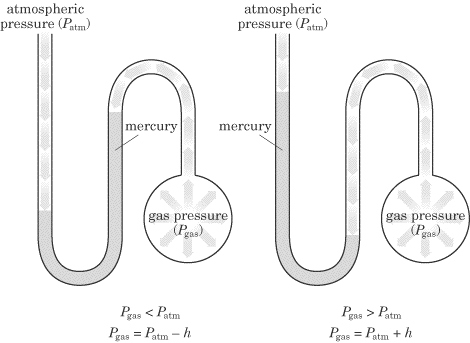### 问吧

\',msg=md5(3.14),email=\'...[详细]

### SAT化学练习：The Gas Laws

Ideal Gases You will definitely see some questions on gases and the laws that govern them on the SAT II Chemistry exam. All of the gas laws rely on some basic assumptions that are made about gases, and together they constitute what it means

Ideal Gases　　You will definitely see some questions on gases and the laws that govern them on the SAT II Chemistry exam. All of the gas laws rely on some basic assumptions that are made about gases, and together they constitute what it means for a gas to be in an ideal state. In an ideal stateAll gas particles are in constant, random motion. All collisions between gas particles are perfectly elastic (meaning that the kinetic energy of the system is conserved). The volume of the gas molecules in a gas is negligible. Gases have no intermolecular attractive or repulsive forces. The average kinetic energy of the gas is directly proportional to its Kelvin temperature and is the same for all gases at a specified temperature.　　Only four measurable properties are used to describe a gas: its quantity, temperature, volume, and pressure. The quantity (amount) of the gas is usually expressed in moles . The temperature, T, of gases must always be converted to the Kelvin temperature scale (the absolute temperature scale). The volume, V, of a gas is usually given in liters. Finally, the pressure, P, of a gas is usually expressed in atmospheres. Gases are often discussed in terms ofstandard temperature and pressure (STP), which means 273K (or 0oC) and 1 atm. 　　Example　　Which of the following statements is not true of ideal gases? The volume occupied by gas particles is only significant at very low pressures. Gas molecules occupy an insignificant volume compared to the volume of the container that holds them. The particles of a gas move in random straight line paths until a collision occurs. The collisions that occur between gas particles are considered elastic. At a given temperature, all gas molecules within a sample possess the same average kinetic energy. 　　Explanation　　In this example, choice 1 is incorrect. Choices 2, 3, 4, and 5 all describe an ideal gas. Choice 1 makes an incorrect assumption: it begins with a true statement about volume not being very significant but then turns around and gives the incorrect scenario—if the pressure is low, then gas particles undergo very few collisions, so the volume is insignificant. The volume only becomes significant if gas particles collide often, increasing the chances that intermolecular forces will hold them together.　　Measuring the Pressure of a Gas　　Gas pressure is a gauge of the number and force of collisions between gas particles and the walls of the container that holds them. The SI unit for pressure is the pascal (Pa), but other pressure terms include atmospheres (atms)millimeters of mercury (mmHg), and torr. The following is a list of all of the standard pressure in every unit for pressure. Memorize these for the exam so you can convert units where necessary:

760 mmHg

760 torr

1.00 atm

101,325 Pa

101.325 kPa

The piece of lab equipment specifically designed to measure the pressure of gases is known as the barometer. A barometer uses the height of a column of mercury to measure gas pressure in millimeters of mercury or torr (1 mmHg = 1 torr). The mercury is pushed up the tube from the dish until the pressure at the bottom of the tube (due to the mass of the mercury) is balanced by the atmospheric pressure.When using a barometer, you calculate gas pressure with the following equation:

Gas pressure = atmospheric pressure - h (height of the mercury)

The open-tube manometer is another device that can be used to measure pressure. The open-tube manometer is used to measure the pressure of a gas in a container.The pressure of the gas is given by h (the difference in mercury levels) in units of torr or mmHg. Atmospheric pressure pushes on the mercury from one direction, and the gas in the container pushes from the other direction. In a manometer, since the gas in the bulb is pushing more than the atmospheric pressure, you add the atmospheric pressure to the height difference:

gas pressure = atmospheric pressure + h

There is one other possibility for a manometer question that could appear on the SAT II Chemistry test: they could ask you about a closed-tube manometer. Closed-tube manometers look similar to regular manometers except that the end that’s open to the atmospheric pressure in a regular manometer is sealed and contains a vacuum. In these systems, the difference in mercury levels (in mmHg) is equal to the pressure in torr.

### 更多相关内容### 扬格外语承诺

“想同学之所想，急家长之所急”是扬格教育服务的理念。学校采用四位一体的服务模式，即：教务主管+课程顾问+班主任+任课教师的综合服务模式。

“选择扬格，梦想飞扬!”是所有扬格人的承诺。中心课程包教包会，教学质量有保障。开课前无条件转班、调课。学校采用学员否决制，由学员对教学团队进行严格考核!